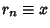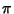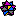## Base (Number)

A Real Numbercan be represented using any Integer numberas a base (sometimes also called a Radix or Scale). The choice of a base yields to a representation of numbers known as a Number System. In base, the Digits 0, 1, ...,are used (where, by convention, for bases larger than 10, the symbols A, B, C, ...are generally used as symbols representing the Decimal numbers 10, 11, 12, ...).

 Base Name 2 Binary 3 Ternary 4 Quaternary 5 Quinary 6 Senary 7 Septenary 8 Octal 9 Nonary 10 Decimal 11 Undenary 12 Duodecimal 16 Hexadecimal 20 Vigesimal 60 Sexagesimal

Let the baserepresentation of a numberbe written(1)

(e.g.,), then the index of the leading Digit needed to represent the number is(2)

whereis the Floor Function. Now, recursively compute the successive Digits(3)

whereand(4)

for,, ..., 1, 0, .... This gives the baserepresentation of. Note that ifis an Integer, thenneed only run through 0, and that ifhas a fractional part, then the expansion may or may not terminate. For example, the Hexadecimal representation of 0.1 (which terminates in Decimal notation) is the infinite expression.

Some number systems use a mixture of bases for counting. Examples include the Mayan calendarand the old British monetary system (in which ha'pennies, pennies, threepence, sixpence, shillings, half crowns, pounds, and guineas corresponded to units of 1/2, 1, 3, 6, 12, 30, 240, and 252, respectively).

Knuth has considered using Transcendental bases. This leads to some rather unfamiliar results, such as equatingto 1 in base,''.

References

Abramowitz, M. and Stegun, C. A. (Eds.). Handbook of Mathematical Functions with Formulas, Graphs, and Mathematical Tables, 9th printing. New York: Dover, p. 28, 1972.

Bogomolny, A. Base Converter.'' http://www.cut-the-knot.com/binary.html.

Lauwerier, H. Fractals: Endlessly Repeated Geometric Figures. Princeton, NJ: Princeton University Press, pp. 6-11, 1991.Weisstein, E. W. Bases.'' Mathematica notebook Bases.m.